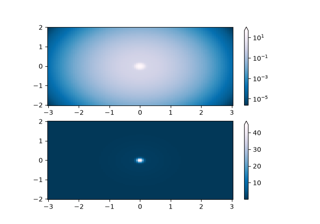# matplotlib.colors.CenteredNorm#

class matplotlib.colors.CenteredNorm(vcenter=0, halfrange=None, clip=False)[source]#

Bases: `Normalize`

Normalize symmetrical data around a center (0 by default).

Unlike `TwoSlopeNorm`, `CenteredNorm` applies an equal rate of change around the center.

Useful when mapping symmetrical data around a conceptual center e.g., data that range from -2 to 4, with 0 as the midpoint, and with equal rates of change around that midpoint.

Parameters:
vcenterfloat, default: 0

The data value that defines `0.5` in the normalization.

halfrangefloat, optional

The range of data values that defines a range of `0.5` in the normalization, so that vcenter - halfrange is `0.0` and vcenter + halfrange is `1.0` in the normalization. Defaults to the largest absolute difference to vcenter for the values in the dataset.

clipbool, default: False

Determines the behavior for mapping values outside the range `[vmin, vmax]`.

If clipping is off, values outside the range `[vmin, vmax]` are also transformed, resulting in values outside `[0, 1]`. For a standard use with colormaps, this behavior is desired because colormaps mark these outside values with specific colors for over or under.

If `True` values falling outside the range `[vmin, vmax]`, are mapped to 0 or 1, whichever is closer. This makes these values indistinguishable from regular boundary values and can lead to misinterpretation of the data.

Examples

This maps data values -2 to 0.25, 0 to 0.5, and 4 to 1.0 (assuming equal rates of change above and below 0.0):

```>>> import matplotlib.colors as mcolors
>>> norm = mcolors.CenteredNorm(halfrange=4.0)
>>> data = [-2., 0., 4.]
>>> norm(data)
array([0.25, 0.5 , 1.  ])
```
autoscale(A)[source]#

Set halfrange to `max(abs(A-vcenter))`, then set vmin and vmax.

autoscale_None(A)[source]#

Set vmin and vmax.

property halfrange#
property vcenter#
property vmax#
property vmin#

## Examples using `matplotlib.colors.CenteredNorm`#Colormap Normalization

Colormap Normalization